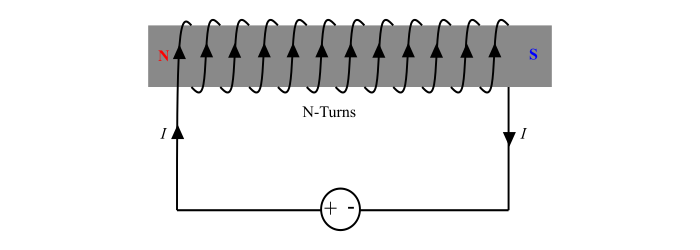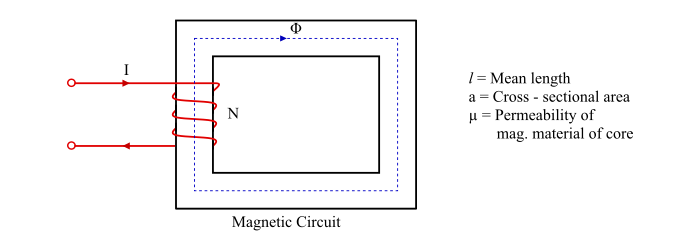# What is Magnetomotive Force (MMF)?

The Magnetomotive force (MMF) is defined as the work done in moving a unit magnetic pole once around the magnetic circuit. It is a magnetic pressure that tends to set up magnetic flux in a magnetic circuit. Mathematically, the mmf is the product of current and number of turns of a coil.

Consider the coil consisting of N turns and an electric current of I amperes flowing in it. Therefore, the mmf is given by,Magnetomotive force, MMF = 𝑁𝐼 … (1)

## Unit of MMF

Since MMF is a product of current in amperes (A) and number of turns (T) in a coil, thus

Unit of MMF = Ampere − Turns (or AT)

## MMF Formulae• In any magnetic circuit, if H being the magnetising force and l is the length of the magnetic circuit, then the mmf for the magnetic circuit is given by,

MMF = 𝐻 × 𝑙 … (2)

• In any magnetic circuit, if Φ being the magnetic flux and S is the magnetic reluctance of the magnetic circuit. Then, the mmf required is given by,

$$\mathrm{MMF=Magnetic\:flux(\phi)\times\:Reluctance(S)}$$

$$\mathrm{\Rightarrow\:MMF=\phi\:\times\:\frac{l}{a\mu_{0}\mu_{r}}=\frac{\phi}{a}\times\:\frac{1}{\mu_{0}\mu_{r}}}$$

$$\mathrm{(\because\:S=\frac{1}{\mu_{0}\mu_{r}}\:and\:\frac{\phi}{a}=B(Magnetic\:flux\:density))}$$

$$\mathrm{\therefore\:MMF=B\times\:\frac{1}{\mu_{0}\mu_{r}}=H\times\:l\:\:\:...(3)}$$

$$\mathrm{(\because\:H=\frac{B}{\mu_{0}\mu_{r}})}$$

## Numerical Example (1)

An electromagnet consists of a coil of 1500 turns, if 2 A current flows in the coil. Determine the mmf of the electromagnet.

Solution

MMF = NI = 1500 × 2 = 3000 AT

## Numerical Example (2)

A magnetic flux density of 1.6 Wb/m2 is required in the air gap of 4 mm. Calculate the mmf required.

Solution

$$\mathrm{Magnetising\:force,H=\frac{B}{\mu_{0}}=\frac{1.6}{4\pi\times\:10^{-7}}1.273\times\:10^{6}\:AT/m}$$

$$\mathrm{\therefore\:MMF\:required=H\times\:l=(1.273\times\:10^{6})\times\:(4\times\:10^{-3})}$$

$$\mathrm{\Rightarrow\:MMF\:required=5092\:AT}$$